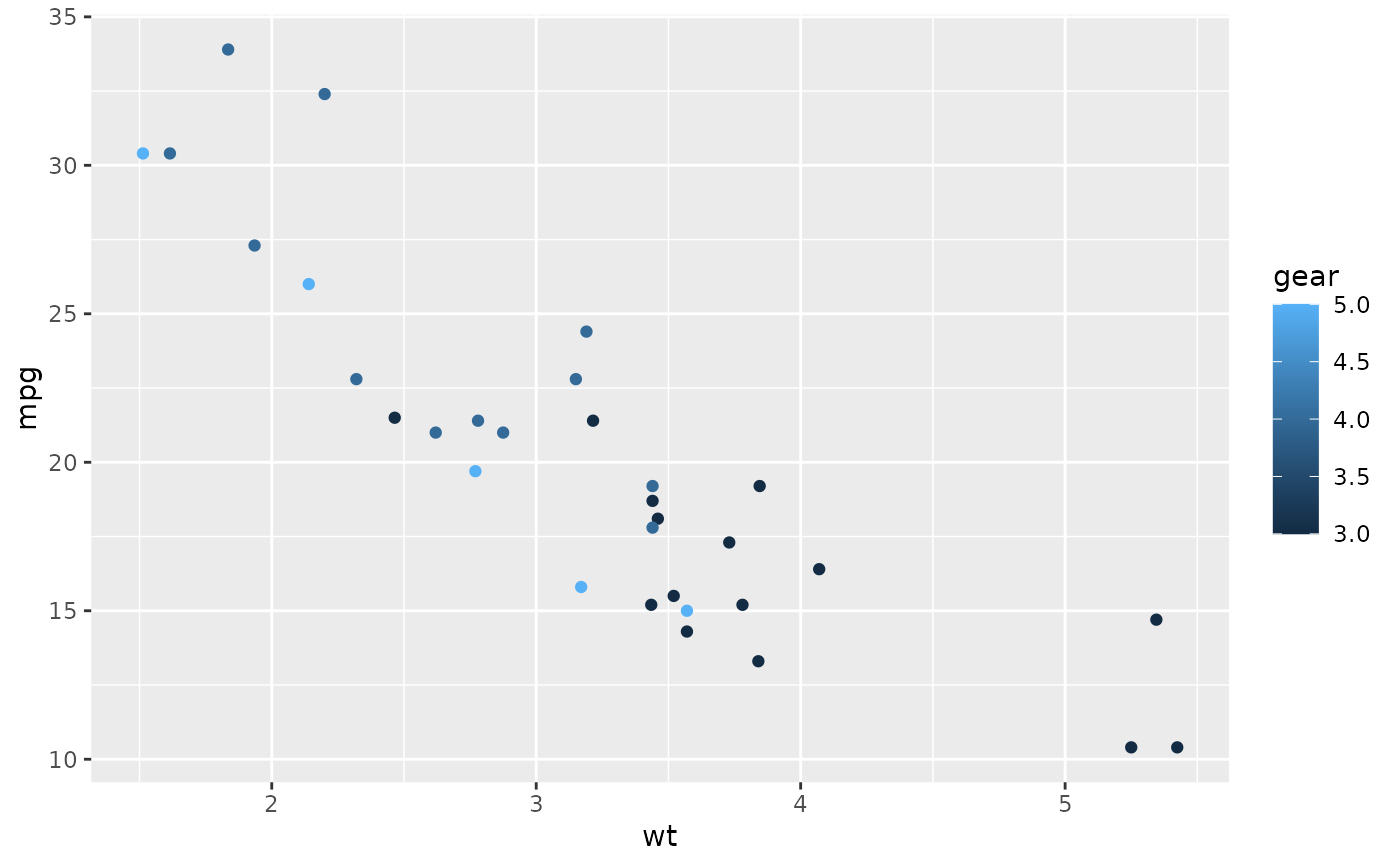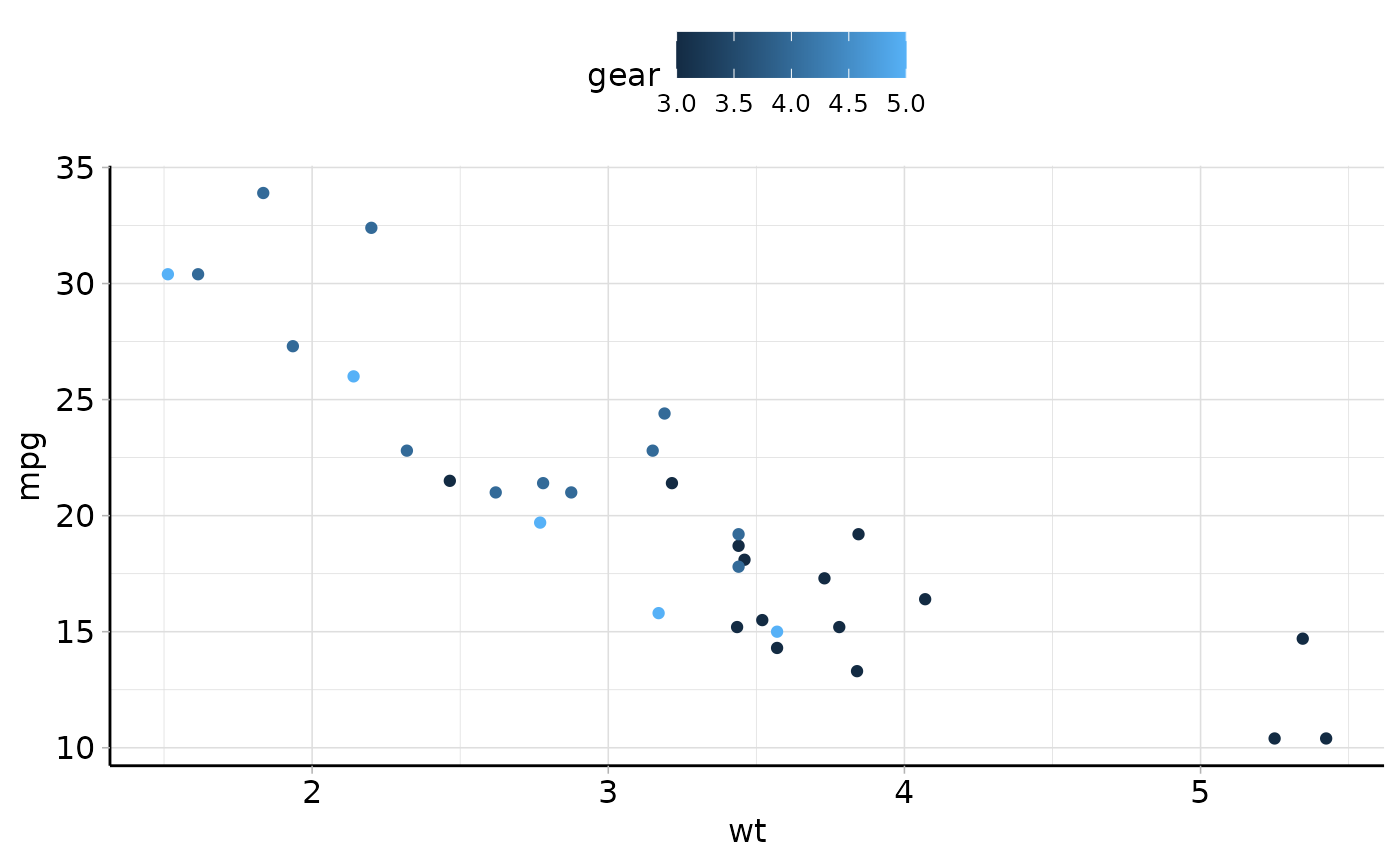The theme is heavily influenced and partly based on ggpubr::theme_pubr(). This theme is applied by default to all autoplot() methods in the mlr3 ecosystem. If you do not like it and want to use the default ggplot2 theme, you can add + theme_gray() to the autoplot() call.

## Usage

theme_mlr3(
base_size = 12,
base_family = "",
border = FALSE,
margin = TRUE,
legend = c("top", "bottom", "left", "right", "none"),
x.text.angle = 0
)

## Arguments

base_size

[integer]
Text font size.

base_family

[character]
Font family.

border

[logical]
If TRUE, adds a panel border.

margin

[logical]
If FALSE, reduces the plot margin(s).

legend

[character]
Specifies the legend position. Allowed values are one of c("top", "bottom", "left", "right", "none"). Default is "top".

x.text.angle

[numeric]
Rotation angle of x axis tick labels. Default value is 0. Use 90 for vertical text.

## Examples

library("ggplot2")
p = ggplot(mtcars, aes(x = wt, y = mpg)) +
geom_point(aes(color = gear))

# Default plot
p# theme_mlr3()
p + theme_mlr3()